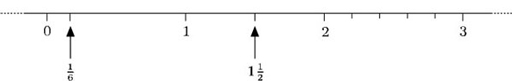Numbers, units and arithmetic

This free course is available to start right now. Review the full course description and key learning outcomes and create an account and enrol if you want a free statement of participation.

Free course

# 1.8 Simplest form of a fraction

The fraction, is the simplest form of all its equivalent fractions, because it cannot be ‘simplified’ further (by dividing top and bottom by the same whole number called a common factor);or, on the other hand, can be simplified toby dividing top and bottom by 2 and 4 respectively.

A fraction is in its simplest form if the numerator and the denominator have no common factors.

6 can be written as 2 × 3. 2 and 3 are called factors of 6.

15 can be written as 5 × 3. 5 and 3 are factors of 15.

3 is a common factor of 6 and 15. Hence. This is the simplest form of.

## Example 6

• (a) Find some equivalent fractions for.

• (b) What is the simplest form of?

Multiply or divide top and bottom by the same whole number to give an equivalent fraction.

• (a) Some examples are• (b) Top and bottom are divisible by 5. So3 and 4 have no common factors sois the simplest form.

You can indicate fractions along the number line by marking points between the whole numbers. The numbersandare shown below.Notemay also be written as 1.5 or as.

MU120_4M1